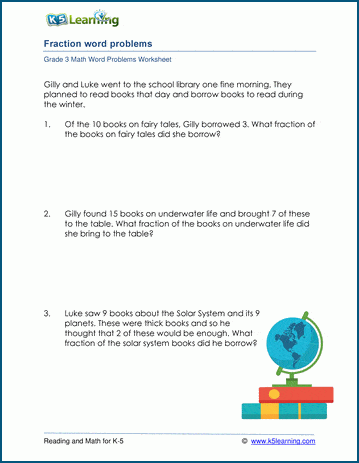# 3rd Grade Fraction Word Problems Worksheet

## Fraction word problem worksheets

These grade 3 worksheets give a selection of word problems dealing with fractions.  The material is introductory level and is intended to highlight the use of fractions in real life situations.

## Identifying and comparing fractions word problems

These printable worksheets have grade 3 word problems related to identifying and/or comparing fractions. They also provide practice in simplifying fractions.  Both fractions and mixed numbers are used.

## Adding and subtracting fractions word problems

These worksheets have word problems requiring simple addition and subtraction of fractions and mixed numbers.  Only fractions with like denominators are considered.Sample Grade 3 Fraction Word Problem Worksheet

## More word problem worksheets

Explore all of our math word problem worksheets, from kindergarten through grade 5.

## What is K5?

K5 Learning offers reading and math worksheets, workbooks and an online reading and math program for kids in kindergarten to grade 5.  We help your children build good study habits and excel in school.